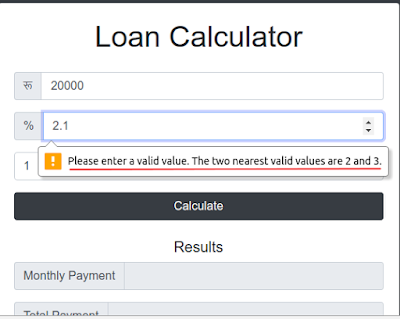## Monday, April 23, 2018

### [SOLVED] - How to Enter Floating-Point/Decimal Numbers in HTML5 Forms

If you have a form where you have declare a input type as below:
`````` <input type="number" name="quantity" min="1" max="5">
``````

Now if you enter a floating-point/decimal value like '2.1' and try to process your form then you will get the following error message:

"Please enter a valid value. The two nearest valid values are 2 and 3."Click on image to enlarge
Solution:
step="any"
`````` <input type="number" name="quantity" min="1" max="5" step="any">